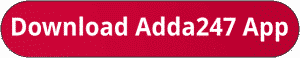Latest Banking jobs   »   IBPS quant

# Quantitative Aptitude Quiz For IBPS Clerk Mains 2023-13th October

Directions (1-5): Study the following graphs carefully and answer the questions given below:
Graph given below shows the monthly expenditure by 6 personsGiven below is the pie chart for percentage breakup of monthly Income of person B.
Note- Income = Expenditure + SavingQ1. If saving of C is equal to the sum of expenditure of E on clothing and bill together and expenditure of E on Bill is 25% less than expenditure of B on bill then what is the sum of savings of C and B together, given that the ratio of expenditure of E on clothing and Bill is 5 : 3.
(a) 13250
(b) 14400
(c) 15550
(d) 12560
(e) None of these
Q2. If expenditure of A and C on clothing is 25% and 15% respectively of their expenditure and saving of A is 25% more than expenditure of A on clothing, then sum of saving of A and expenditure of C on clothing together is what percent of expenditure of B on clothing?
(a) 155%
(b) 180.5%
(c) 160.75%
(d) 166.67%
(e) 200%Directions (6-10): In each of these questions, two equations (I) and (II) are given. You have to solve both the equations and give answer
(a) if x>y
(b) if x≥y
(c) if x<y
(d) if x ≤y
(e) if x = y or no relation can be established between x and y.Solutions. .               .                                      .       .

## FAQs

### When is the IBPS Clerk Mains exam scheduled for?

The IBPS Clerk Mains exam is scheduled for 7 October 2023.

#### Congratulations!Union Budget 2023-24: Free PDF